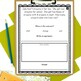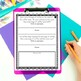# 2nd Grade Word Problem of the Day Story Problems- BundleSubject
Resource Type
Format
Zip (12 MB|288 pages)
Standards
\$21.00
Bundle
List Price:
\$27.00
You Save:
\$6.00
\$21.00
Bundle
List Price:
\$27.00
You Save:
\$6.00
Products in this Bundle (10)

showing 1-5 of 10 products

• Bundle Description
• Standards

These Problem of the Day word problems contain real-world applications of the second grade math standards. This nine month 2nd Grade Word Problem bundle includes 30 story problems for each month of the school year (Sept-May) that build students' problem solving skills through guided and independent practice of the standards daily. These rigorous, multi-step Problem of the Day word problems are guided and independent practice pages to give students practice and exposure with varying levels of difficulty.

The units begin with students identifying if the unknown is at the start, change, or the end only working with numbers within 20. It continues to scaffold and build throughout the year leading up to students working with addition and subtraction with and without regrouping, counting coins in word problems, working with time, arrays, and fractions in real world formats, and working with numbers beyond 1,000. April and May include all multi-step problems increasing the rigor of students' practice.

SKILLS INCLUDED:

• facts within 20

• subtraction without regrouping

• subtraction with regrouping

• place value

• money

• part-part-whole tables

• start-change-end tables

• drawing pictures to model the problem

• length word problems

• visual models of area and fractions

• multi-step problems

Some pages can be completed by students independently while others are intended for students to experience a productive struggle as they work to apply what they know. The purpose of varying the difficulty of the skills is to build confidence in students’ problem solving strategies, while also pushing them to try new strategies independently. Some problems include many steps to reach the solution while others require students to explain their work.

The sets provide enough pages so that a page can be completed each day, if you wish, with additional pages going home for independent practice. These can be easily printed and stapled for daily use, or these are also perfect for inclusion in interactive notebooks.

The other 2nd Grade Word Problems of the Day:

Sept | Oct | Nov | Dec | Jan | Feb | March | April | May

Or, try out the Back to School Freebie.

CLICK HERE to follow me and be notified of future products as soon as they are posted.

_______________________________________________________________

You may not redistribute, edit, sell, or otherwise post this product on the internet. You may, however, post a link for others topurchase themselves.

to see state-specific standards (only available in the US).
Look for and express regularity in repeated reasoning. Mathematically proficient students notice if calculations are repeated, and look both for general methods and for shortcuts. Upper elementary students might notice when dividing 25 by 11 that they are repeating the same calculations over and over again, and conclude they have a repeating decimal. By paying attention to the calculation of slope as they repeatedly check whether points are on the line through (1, 2) with slope 3, middle school students might abstract the equation (𝑦 – 2)/(𝑥 – 1) = 3. Noticing the regularity in the way terms cancel when expanding (𝑥 – 1)(𝑥 + 1), (𝑥 – 1)(𝑥² + 𝑥 + 1), and (𝑥 – 1)(𝑥³ + 𝑥² + 𝑥 + 1) might lead them to the general formula for the sum of a geometric series. As they work to solve a problem, mathematically proficient students maintain oversight of the process, while attending to the details. They continually evaluate the reasonableness of their intermediate results.
Look for and make use of structure. Mathematically proficient students look closely to discern a pattern or structure. Young students, for example, might notice that three and seven more is the same amount as seven and three more, or they may sort a collection of shapes according to how many sides the shapes have. Later, students will see 7 × 8 equals the well remembered 7 × 5 + 7 × 3, in preparation for learning about the distributive property. In the expression 𝑥² + 9𝑥 + 14, older students can see the 14 as 2 × 7 and the 9 as 2 + 7. They recognize the significance of an existing line in a geometric figure and can use the strategy of drawing an auxiliary line for solving problems. They also can step back for an overview and shift perspective. They can see complicated things, such as some algebraic expressions, as single objects or as being composed of several objects. For example, they can see 5 – 3(𝑥 – 𝑦)² as 5 minus a positive number times a square and use that to realize that its value cannot be more than 5 for any real numbers 𝑥 and 𝑦.
Attend to precision. Mathematically proficient students try to communicate precisely to others. They try to use clear definitions in discussion with others and in their own reasoning. They state the meaning of the symbols they choose, including using the equal sign consistently and appropriately. They are careful about specifying units of measure, and labeling axes to clarify the correspondence with quantities in a problem. They calculate accurately and efficiently, express numerical answers with a degree of precision appropriate for the problem context. In the elementary grades, students give carefully formulated explanations to each other. By the time they reach high school they have learned to examine claims and make explicit use of definitions.
Use appropriate tools strategically. Mathematically proficient students consider the available tools when solving a mathematical problem. These tools might include pencil and paper, concrete models, a ruler, a protractor, a calculator, a spreadsheet, a computer algebra system, a statistical package, or dynamic geometry software. Proficient students are sufficiently familiar with tools appropriate for their grade or course to make sound decisions about when each of these tools might be helpful, recognizing both the insight to be gained and their limitations. For example, mathematically proficient high school students analyze graphs of functions and solutions generated using a graphing calculator. They detect possible errors by strategically using estimation and other mathematical knowledge. When making mathematical models, they know that technology can enable them to visualize the results of varying assumptions, explore consequences, and compare predictions with data. Mathematically proficient students at various grade levels are able to identify relevant external mathematical resources, such as digital content located on a website, and use them to pose or solve problems. They are able to use technological tools to explore and deepen their understanding of concepts.
Model with mathematics. Mathematically proficient students can apply the mathematics they know to solve problems arising in everyday life, society, and the workplace. In early grades, this might be as simple as writing an addition equation to describe a situation. In middle grades, a student might apply proportional reasoning to plan a school event or analyze a problem in the community. By high school, a student might use geometry to solve a design problem or use a function to describe how one quantity of interest depends on another. Mathematically proficient students who can apply what they know are comfortable making assumptions and approximations to simplify a complicated situation, realizing that these may need revision later. They are able to identify important quantities in a practical situation and map their relationships using such tools as diagrams, two-way tables, graphs, flowcharts and formulas. They can analyze those relationships mathematically to draw conclusions. They routinely interpret their mathematical results in the context of the situation and reflect on whether the results make sense, possibly improving the model if it has not served its purpose.
Total Pages
288 pages
N/A
Teaching Duration
1 Year
Report this Resource to TpT
Reported resources will be reviewed by our team. Report this resource to let us know if this resource violates TpT’s content guidelines.
• Ratings & Reviews
• Q & A

Teachers Pay Teachers is an online marketplace where teachers buy and sell original educational materials.